Courses

# Revisal Problems For JEE Main (Liquid Solutions)

## 24 Questions MCQ Test Chemistry for JEE Advanced | Revisal Problems For JEE Main (Liquid Solutions)

Description
This mock test of Revisal Problems For JEE Main (Liquid Solutions) for JEE helps you for every JEE entrance exam. This contains 24 Multiple Choice Questions for JEE Revisal Problems For JEE Main (Liquid Solutions) (mcq) to study with solutions a complete question bank. The solved questions answers in this Revisal Problems For JEE Main (Liquid Solutions) quiz give you a good mix of easy questions and tough questions. JEE students definitely take this Revisal Problems For JEE Main (Liquid Solutions) exercise for a better result in the exam. You can find other Revisal Problems For JEE Main (Liquid Solutions) extra questions, long questions & short questions for JEE on EduRev as well by searching above.
QUESTION: 1

### Only One Option Correct Type This section contains 22 multiple choice questions. Each question has four choices (a), (b), (c) and (d), out of which ONLY ONE is correct Q. The partial pressure of ethane over a solution containing 6.56x 10-3 g of ethane is 1 bar. If the solution contains 5.00x 10-2 g ethane, then partial pressure

Solution: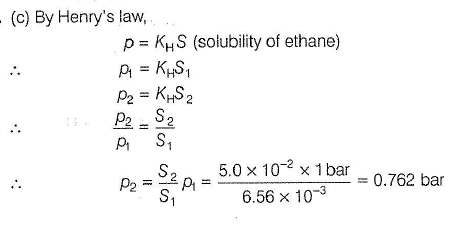QUESTION: 2

### Concentrated HN03 is 63% by m ass in a queous solution and having density 1.5 g mL-1. Molarity of a solution obtained by diluting 10 mL of this HN03 to 100 mL solution is

Solution:

(a) HN03 is 63 % by mass, thus

100 g of HN03 solution has HN03 = 63 g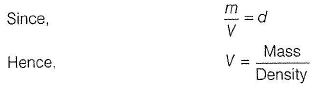Hence, 100/15 m L of HN03 solution has HN03 = 63 g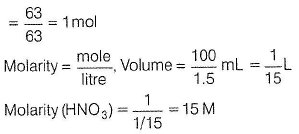10 mL of this solution is diluted to 100 mL.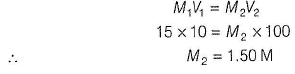QUESTION: 3

### Two elements A and B form compounds AB2 and AB4 (both are non-volatile and non-electrolyte) when dissolved in 20.0 g benzene, 1 g of AB2 lowers the freezing point of benzene by 2.3 K, whereas 1.0 g of AB4 lowers it by 1.3 K [Kf (benzene) = 5.5 K mol-1 kg]. ; Thus, atomic masses of A and B respectively, ar

Solution:

w1/m1= moles of solute in w2 g solvent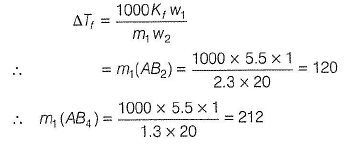A+2B =120                       ...(i)

A+4B = 212                   ...(2)

On solving Eqs. (i) and (Hi), we get

∴       B = 46
A = 28

QUESTION: 4

Vapour pressure of pure water at 298 K is 23.8 mm. Thus, vapour pressure of 20% glucose ! solution (weight/volume of solution) is (density of solution is 0.9971 g/cc

Solution:

(d) 100 cc of solution has glucose = 20 g 100 x 0.9971 g of solution has glucose = 20 g 99.71 g of solution has glucose = 20 g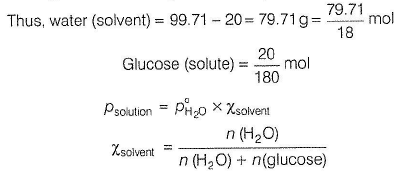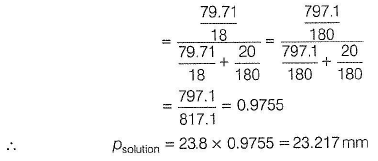QUESTION: 5

Pure and dry N2 gas was bubbled through a solution of 2.25 g of a non-volatile solute A in 150 g benzene and through pure benzene.
Loss in weight of solution = 2.154 g and loss in weight of benzene = 0.160 g Thus, molar mass of solute is

Solution: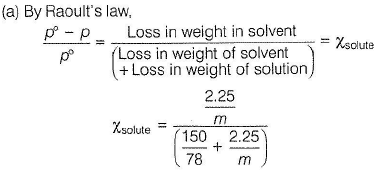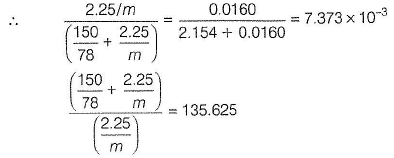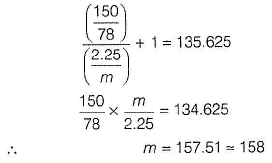QUESTION: 6

Mass of methyl alcohol (CH3OH) which when dissolved in 100 g of water would just prevent formation of ice at - 9.3° C is [Kf (H20 ) = 1.860° mol-1 kg]

Solution: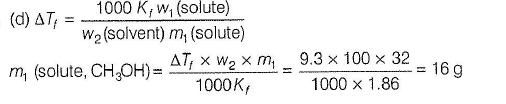QUESTION: 7

At 27° C, an aqueous solution of a carbohydrate containing 18 g per 100 mL of the solution is found to be isotonic with aqueous solution containing 2.925 g NaCI per 100 mL of the solution. Thus, molar mass of the carbohydrate is (assume NaCI is completely ionised)

Solution:

(b) For carbohydrate,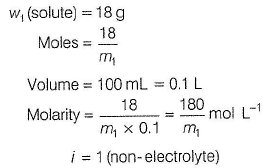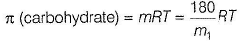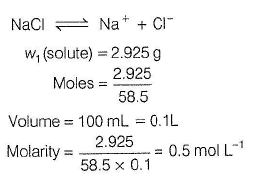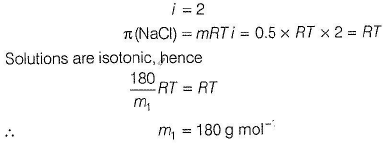QUESTION: 8

Mass percentage of the resultant solution obtained by mixing 300 g of 25% solution and 400 g of 40% solution by mass is

Solution: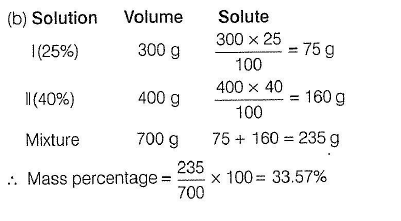QUESTION: 9

1 g mixture of Na2C03 and NaHC03 each having in 1 : 1 m olar ratio required x mL of 0.1 M HCI for complete reaction. What is the value of x?

Solution: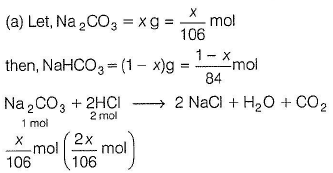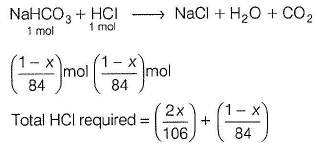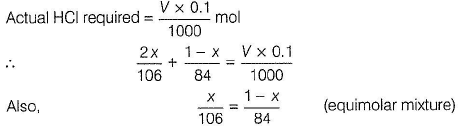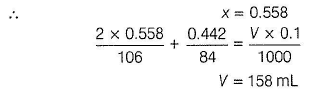QUESTION: 10

The partial molar volumes of propanone (CH3COCH3)and butanone (CH3COCH2CH3) in a mixture in which mole fraction of propanone is 0.4 are   75 cm3 mol-1 and 80 cm3 mol-1. Thus, the total volume of solution of total mass 1 kg is

Solution: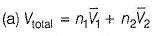where,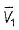and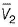are molar volumes and n1, and n2 are fiyfhber of moles.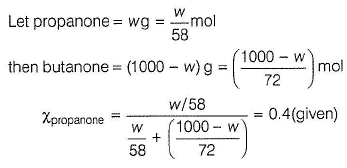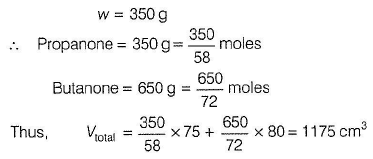QUESTION: 11

Correct order of boiling point of the following a queous solution is (assume molarity = molality)

I. 0.01 M Na2S04,

II. 0.01 M Na3P04,

III. 0.01 M NaBr,

IV. 0.01 M glucose solution

Solution:

(c) ΔTb = molality x Kb x i

Solutions are equimolar in same solvent, thus ΔTb ∝ i

Also, boiling poin t of the solution = (boiling point of H2O + ΔTb)

Thus, greater the value of i, larger the boiling point, x = degree of ionisation.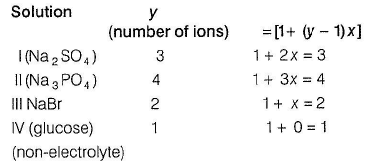Thus, van’t Hoff factor is in the order, IV < III < I < II and boiling point is in the order, IV < III < I < II.

QUESTION: 12

The vapour pressure of an aqueous solution of CaCI2 at 25°C is 0.02970 atm. The vapour pressure of water at the same temperature is 0.03126 atm. Thus, depression in freezing point of the solution is [Kf (H20) = 1.86° mol-1 kg]

Solution: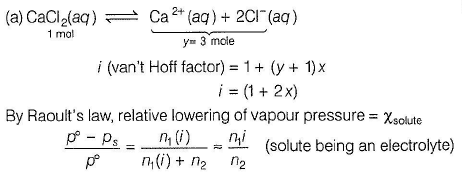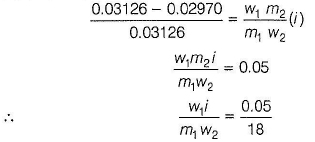Depression in freezing point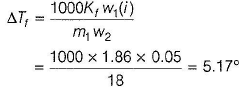QUESTION: 13

If sodium sulphate is considered to be completely dissociated into cations and anions in aqueous solution, the change in freezing point of water (ΔTf ), when 0.01 mole of sodium sulphate is dissolved in 1 kg of water is [Kf (H20) = 1.86° mol-1 kg]

Solution: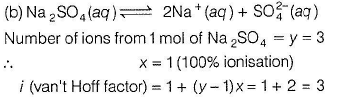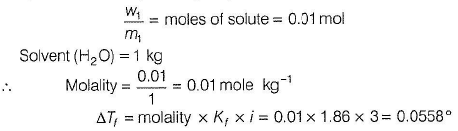QUESTION: 14

122 g of benzoic acid is dissolved in 1000 g of benzene at 10°C at which vapour pressure of benzene is 66.6 cm of Hg. If benzoic acid is completely dimerised in benzene, then vapour pressure of the solution of the benzoic acid in benzene is

Solution:

(c) There is dim er formation of benzoic acid in benzene due to intermolecular H-bonding.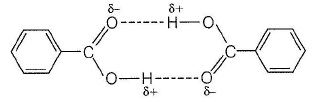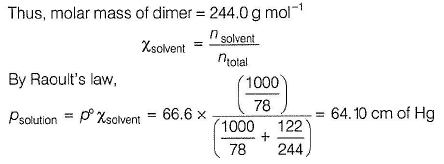QUESTION: 15

Osmotic pressure of decimolar potassium ferrocyanide solution which is 40% ionised at 300 K is

Solution:

(c) Potassium ferrocyanide is K4[Fe(CN)6] .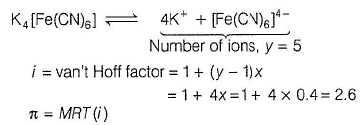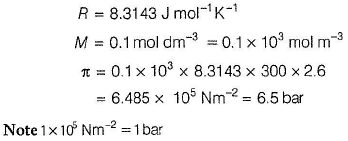QUESTION: 16

How many milliliters o f glycerol (C3H803) of d ensity 1.26 g mL-1 m ust be a dd ed per kilogram of water to produce a solution with 4.85 mol % of C3H8O3?

Solution: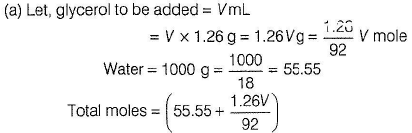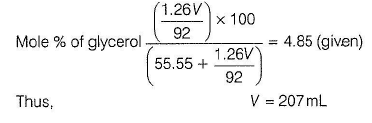QUESTION: 17

A beaker containing 0,010 mol of C12H22O11 in 100 g H2O and a beaker  containing .020 mol of C12H22O11 are placed in a chamber and allowed to equilibrate. Thus, mole fraction of  C12H22O11  in both solutions is

Solution:

(a) Water vapour will be transferred from the more dilute solution to the more concentrated solution until both solutions reach at the same concentration.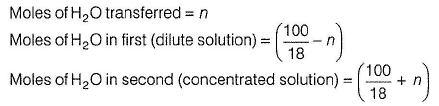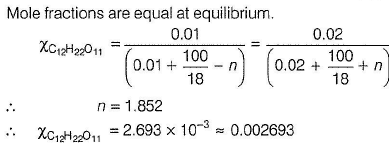QUESTION: 18

The vapour pressure of pure benzene at a certain temperature is 640 mm Hg. A non-volatile, non-electrolyte solid weighing 2.175 g is added to 39.0 g of benzene. The vapour pressure of the solution is 600 mm Hg. Molecular weight of the non-electrolyte is

Solution: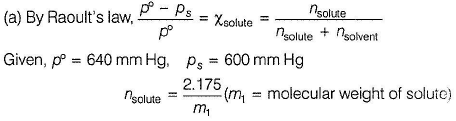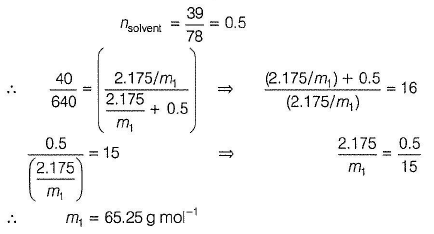QUESTION: 19

For a dilute solution containing 2.5 g of a non-volatile, non-electrolyte solute in 100 g of water, the elevation in boiling point at 1 atm is 2°C. Assuming concentration of the solute is much lower than that of solvent, vapour pressure (mm of Hg) of the solution is (Kb =0.76 K kg mol-1

Solution: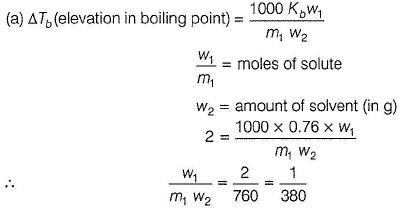By Raoult’s law for dilute solution,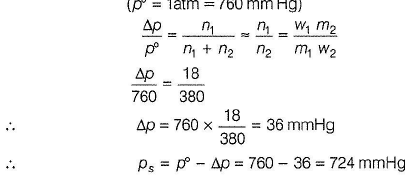QUESTION: 20

Henry’s law constant for C02 in water is 1,67x 108 Pa at 298 K. C02 gas is packed in 500 mL H2O at 298 K under a pressure of 2.5 atm. Thus, quantity of C02 in 500 mL H20 is

Solution: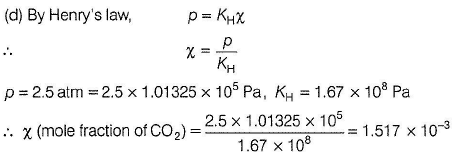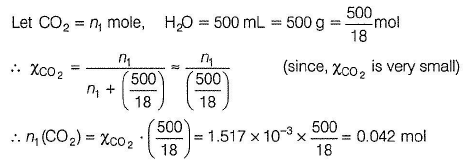QUESTION: 21

Consider the separate solutions of

0.500 M C2H5OH (aq)

0.100 M Mg3(P04)2(aq)

0.250 M KBr(ag)

0.125 MNa3PO4(aq)

each at 25°C.
Which statement is true about these solutions assuming all salts to be strong electrolytes?

[JEE Main 2014]

Solution:

(a) Salts have been assumed as strong electrolytes, van't Hoff factor, i = [1 + (y - 1)x]

y = number of ions from one mole of salt

x = degree of ionisation = 1

C2H5OH is a non-electrolyte hence, y = 1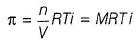Thus,  π ∝ Mi, RT being constant. Greater the value of Mi, greater the osmotic pressure.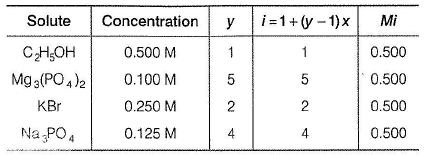Thus, π ∝ Mi have equal values.

QUESTION: 22

For a weak monobasic acid, pKa = 4 then van’t Hoff factor for 0.01 M acid is

Solution: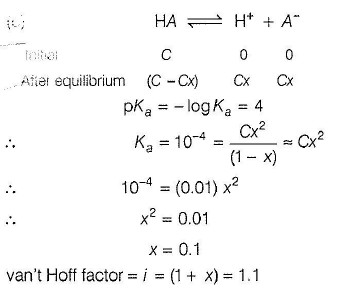QUESTION: 23

Statement Type
This section is based on Statement I and Statement II. Select the correct anser from the codes given below

Q

Statement I : 6% urea solution and 6% acetic acid solution in water are equimolar.

Statement II : Equimolar solutions are isotonic.

Solution: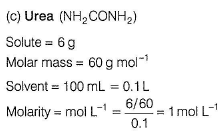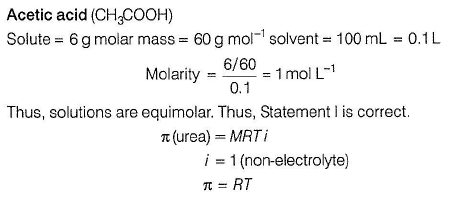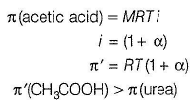Thus, solutions are not isotonic (they have different osmotic pressure. Thus, Statement II is incorrect.

QUESTION: 24

Statement I  : 2 g of benzoic acid (C6H5COOH) in 25.0 g of benzene lowered the freezing point of benzene by 1.62 K. Kf (benzene) = 4.86 K kg mol-1.
Statement II :  Depression in freezing point is a colligative property.

Solution: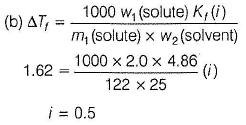In benzene, benzoic acid is dimerised, thus freezing point depression is smaller than expected (/ = 0.5).
ΔTf depends on the number of ions hence, it is a colligative property.
Thus, both statements I and II are correct but Statement II is not the correct explanation of Statement I.